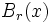The injectivity radius is a scalar function on a Riemannian manifold$M$ is defined as follows: the injectivity radius at$x \in M$ is the supremum of all values$r$ such that the exponential map from the unit ball$B_r(x)$ in$T_xM$, to the manifold$M$, is injective.